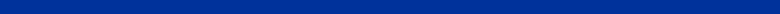CExpression - Expression parser with a Basic-like syntax.
CExpression is an expression parser with a Basic-like syntax which I created for my Visual Scripter project.
It include a lot of standard functions like: AND, OR, XOR, SIN, COS, TAN, LOG, LEN, CHR, ABS, VAL, LEFT, MID, RIGHT, TRIM,
several date and time functions and more.
The class can handle string and numeric variables.
It also includes a validate function that checks the syntax of the expression and returns the error type and position where the error occurred.

Here are some examples of expressions:

Expression: "This" + " " + left\$("iss", 2) + " a test!"
Result: This is a test!

Expression: "The current date is: " + date\$()
Result: The current date is: 08/14/04

Expression: Format\$("It's now: %H:%M:%S")
Result: It's now: 18:55:30

Expression: 10 + val("2" + "2") + abs(-5) + 0.5
Result: 37.5

Expression: len("Pablo") + sqr(4) + max(MyValue,40)
Result: 61

Expression: left\$("Justtttt", 4) + " " + mid\$("aaaa", 2,1) + " "+ right\$("ssssstring", 6)
Result: Just a string

Supported functions:

 AND Logical-and. OR Logical-or. XOR Logical-xor. MOD Divides two numbers and returns only the remainder. NOT Performs logical negation on an expression. SQR Calculates the square root of a number. SQRT Calculates the square root of the given expression. SIN Calculates the sine of an angle. COS Calculates the cosine of an angle. TAN Calculates the tangent of an angle. ARCTAN Calculates the arctangent of an angle. LOG Calculates the natural logarithm of a number. EXP Calculates the exponential. ASC Returns an Numeric representing the character code. LEN Returns the length of a string. ABS Returns the absolute value of a number. SGN Returns the sign of a number. VAL Returns the numbers contained in a string as a numeric value. RANDOM Returns a random number MAX Returns the maximum of two values. MIN Returns the minimum of two values. NOW Returns the current date/time as number. STR\$ Returns a String representation of a number. CHR\$ Returns a String containing the character associated with the specified character code. LEFT\$ Returns a String containing a specified number of characters from the left side of a string. MID\$ Returns a String containing a specified number of characters from a string. RIGHT\$ Returns a String containing a specified number of characters from the right side of a string. TRIM\$ Returns a String containing a copy of a specified string without leading spaces and trailing spaces. LOWER\$ Returns a String containing a copy of a specified string in lower case. UPPER\$ Returns a String containing a copy of a specified string in upper case. DATE\$ Returns the current date as a string. TIME\$ Returns the current time as a string. FORMAT\$ Returns a formatted string, which can be time, date or another valid format expression. + plus - minus * multiply / divide = equal <> not equal >= greater than or equal <= less than or equal > greater than < less than ^ raise a number to the power of an exponent. () grouping© 2015 - Pablo Software SolutionsAll rights reserved.